# Olympiad Test: Lines and Angles

Test Description

## 20 Questions MCQ Test Mathematics (Maths) Class 7 | Olympiad Test: Lines and Angles

Olympiad Test: Lines and Angles for Class 7 2023 is part of Mathematics (Maths) Class 7 preparation. The Olympiad Test: Lines and Angles questions and answers have been prepared according to the Class 7 exam syllabus.The Olympiad Test: Lines and Angles MCQs are made for Class 7 2023 Exam. Find important definitions, questions, notes, meanings, examples, exercises, MCQs and online tests for Olympiad Test: Lines and Angles below.
Solutions of Olympiad Test: Lines and Angles questions in English are available as part of our Mathematics (Maths) Class 7 for Class 7 & Olympiad Test: Lines and Angles solutions in Hindi for Mathematics (Maths) Class 7 course. Download more important topics, notes, lectures and mock test series for Class 7 Exam by signing up for free. Attempt Olympiad Test: Lines and Angles | 20 questions in 20 minutes | Mock test for Class 7 preparation | Free important questions MCQ to study Mathematics (Maths) Class 7 for Class 7 Exam | Download free PDF with solutions
 1 Crore+ students have signed up on EduRev. Have you?
Olympiad Test: Lines and Angles - Question 1

### In a right angled triangle where angle A= 90° and AB=AC. What are the values of angle B.

Detailed Solution for Olympiad Test: Lines and Angles - Question 1

∵ In ∆ABC,
AB = AC
∴ ∠B = ∠C    ...(1)
| Angles opposite to equal sides of a triangle are equal
In ∆ABC,
∠A + ∠B + ∠C = 180°
| Sum of all the angles of a triangle is 180°
⇒ 90° + ∠B + ∠C = 180°
| ∵ ∠A = 90° (given)
⇒    ∠B + ∠C = 90°    ...(2)
From (1) and (2), we get
∠B = ∠C = 45°.

Olympiad Test: Lines and Angles - Question 2

### In figure pair of alternate exterior angles are :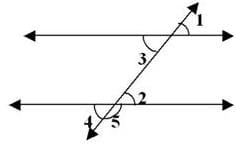Olympiad Test: Lines and Angles - Question 3

### What is the supplement of 105°

Detailed Solution for Olympiad Test: Lines and Angles - Question 3

Supplementary angles sums up to 180° x + 105° = 180° x=180° - 105° = 75°

Olympiad Test: Lines and Angles - Question 4

What is the measure of the complement of 45°?

Detailed Solution for Olympiad Test: Lines and Angles - Question 4

Complementary angle sums up to 90°. So x+ 45° = 90°
x = 90° - 45° = 45°

Olympiad Test: Lines and Angles - Question 5

What is the measure of the complement of 65°?

Olympiad Test: Lines and Angles - Question 6

What is the measure of the complement of 41°?

Detailed Solution for Olympiad Test: Lines and Angles - Question 6

Let the angle be x°
then,complement°=(90-x)°

A/Q,
x=(90-41)
x=90-41
x=49

Olympiad Test: Lines and Angles - Question 7

What is the measure of the supplement of 54°?

Olympiad Test: Lines and Angles - Question 8

Identify which of the following pairs of angles are complementary.

Olympiad Test: Lines and Angles - Question 9

Identify which of the following pairs of angles are supplementary.

Olympiad Test: Lines and Angles - Question 10

Find the angle, which is equal to its complement.

Detailed Solution for Olympiad Test: Lines and Angles - Question 10

Let one of the two equal complementary angles be x.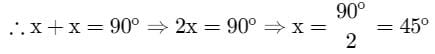Thus, 45is equal to its complement.

Olympiad Test: Lines and Angles - Question 11

Lines l║m, t is transversal then ∠ x ?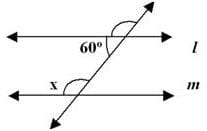Olympiad Test: Lines and Angles - Question 12

Find the angle, which is equal to its supplement.

Detailed Solution for Olympiad Test: Lines and Angles - Question 12

Supplementary angles sums up to 180° since 90° + 90° = 180°, 90° is supplementary to itself

Olympiad Test: Lines and Angles - Question 13

Lines l║m, t is transversal then ∠ x = ?Detailed Solution for Olympiad Test: Lines and Angles - Question 13

120 and x are corresponding angles and corresponding angles are equal so x = 120°

Olympiad Test: Lines and Angles - Question 14

Lines l║m, t is transversal then ∠ z = ?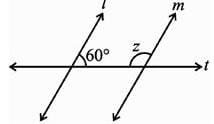Detailed Solution for Olympiad Test: Lines and Angles - Question 14

The angles are co-interior so x+60 = 180
x=120°

Olympiad Test: Lines and Angles - Question 15

Lines l║m, t is transversal then ∠ x = ?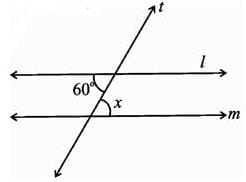Olympiad Test: Lines and Angles - Question 16

ABC is a right angled triangle in which ∠A = 90° and AB = AC. Find ∠B and ∠C.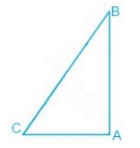Detailed Solution for Olympiad Test: Lines and Angles - Question 16

Given,
∠A = 90° and AB = AC
A/q,
AB = AC
⇒ ∠B = ∠C (Angles opposite to the equal sides are equal.)
Now,
∠A + ∠B + ∠C = 180° (Sum of the interior angles of the triangle.)
⇒ 90° + 2∠B = 180°
⇒ 2∠B = 90°
⇒ ∠B = 45°
Thus, ∠B = ∠C = 45°

Olympiad Test: Lines and Angles - Question 17

The measures of an angle supplement to the angle of 70° is ______.

Olympiad Test: Lines and Angles - Question 18

Find the angle whose measure is five times its complement

Detailed Solution for Olympiad Test: Lines and Angles - Question 18

This will be the equation x=5(90-x)
solution= x=5(90-x)
x+5x=90
6x=90
6x/6=90/6   {divide by 6 to both the side to x}
x=15

ans= The angle is 15 degree and its compliment angle is 75 degreeOlympiad Test: Lines and Angles - Question 19

Find x if I║m.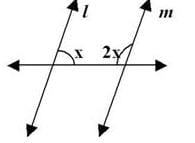Olympiad Test: Lines and Angles - Question 20

Find the value of x if I║m.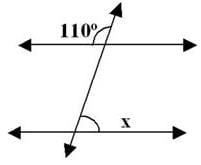## Mathematics (Maths) Class 7

166 videos|276 docs|45 tests
 Use Code STAYHOME200 and get INR 200 additional OFF Use Coupon Code